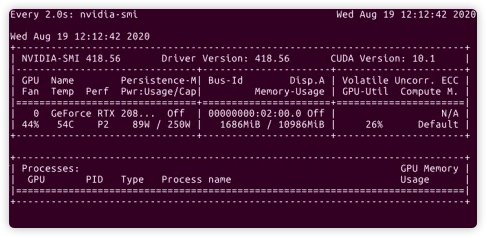# Does the transform operation operate on all data at once or does it operate once every time batch size data is loaded?

For example, I have a set of 10,000 1080P pictures, I resize them to 224 in transform, batch size=20, when the program is running, do I resize 10,000 pictures to 224 at once or resize 20 img at once?

``````train_transform = transforms.Compose(
[transforms.RandomHorizontalFlip(), transforms.RandomCrop(224), transforms.ToTensor(),
transforms.Normalize(mean, std)])
``````

This question is raised because I have 200,000 1080P pictures. If I use 200 of them to train the network, there is no problem, but if I use these 200,000 pictures for training, the graphics card memory will be insufficient.

I want to know how the transform works

They will typically be resized one by one in your dataset class. It’s also possible to do it per batch in the dataloader’s collate function.

200.000 images will not be resized at the same time.

Thank you, can you tell me why the network can run when 200 sheets are used, but not when 10000 sheets are used?

I always thought that the increase in the data set will only increase the training time. If the batch size remains the same, the GPU usage should also remain the same, right?

Yes, you are correct in that the GPU memory usage should remain the same. If it’s increasing for every batch you most likely have a bug in the program.

Do you have such a problem?

Yes, I have this problem. If I use 200 images for training, it will take about 10 seconds for the GPU to use from 1800MB to 4848MB, but if I choose 10,000 images for training, it will take about 1 hour for the GPU to go from 1800MB to out of memory.

Do you know how to solve it ?

Yes I think that I can help you1. Make sure to do the backpropagation. `loss.backward()`
2. Don’t hold on to the image, labels, loss or any other GPU tensor in another list. If you want to save e.g. the loss, save the `loss.item()` so the GPU can free the memory.

If this won’t solve your problem, please post the code of your training loopThank you so much! Yes , I have `loss.backward()`, But I don’t quite understand the second point you said, Below is my training loop code：

``````    def train():
net.train()
loss_avg = 0.0
for batch_idx, (data, target) in enumerate(train_loader):

# forward
output = net(data)

# backward
loss = F.cross_entropy(output, target)
loss.backward()
optimizer.step()

# exponential moving average
loss_avg = loss_avg * 0.2 + float(loss) * 0.8

state['train_loss'] = loss_avg
``````

``````    def train():
net.train()
loss_avg = 0.0
for batch_idx, (data, target) in enumerate(train_loader):
data, target = data.cuda(), target.cuda()

# forward
output = net(data)

# backward
loss = F.cross_entropy(output, target)
loss.backward()
optimizer.step()

# exponential moving average
loss_avg = loss_avg * 0.2 + loss.item() * 0.8

state['train_loss'] = loss_avg
``````

Thanks Reply. I tested it on two GPUs. After changing the train loop, it only took 10 seconds for 200 images to change from 1686MB to 4848MB. The other 10,000 images have not changed until now.And I found that when batch size=2, I increased the training data from 200 to 550. When I turned on the train loop, I found that the first time from 1686 to 4848, `print(batch_idx)` will output 0 ,1,2,274, and then the GPU will become 4848MB. When batch size=4, the output will be 0,1,2,3,137.
I think that GPU memory will increase only when all the data is running.

This should be abnormal, right? I tested other programs and found that one batch will start to occupy the GPU Instead of using the GPU until all batches are loaded

It’s a bit hard for me to understand you. What do you think is abnormal?
The batches are supposed to be processed one by one, not all at the same time.

I mean, the GPU should process one batch of data at a time? But now it seems that in this program, the GPU processes all the input at once, so it’s okay when there are 200 pictures, but when the pictures are 10,000, the memory is exceeded.

You are correct in that it should process one batch at a time. If it doesn’t that’s a problem. Maybe you have a bug in your dataset/dataloader then? It’s hard to say with the information I have